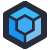Jan's company has an application which needs to handle an Excel spreadsheet, because as I'm fond of pointing out, users love spreadsheets.

The JavaScript code which handles parsing the spreadsheet contains… some choices. These choices caused it to fail on any spreadsheet with more than twenty six columns, and it's not hard to see why.

``````export function generateTableData(worksheet, headerRow) {
const alphabet = ['A','B','C','D','E','F','G','H','I','J','K','L','M','N','O','P','Q','R','S','T','U','V','W','X','Y','Z'
];

// first row is always 1 in the excel (in the loaded data array it is 0)
let cell = '';
let tableData = [];
let emptyRows = 0;

// Running until an empty line is found and breaks
while (currentRow <= 10000) {
let aIndex = 0;
let newEntry = {};
const cell_id = alphabet[aIndex] + currentRow;

// Running Excel columns from a-z. Needs adjustments for more columns!!!!!
while(alphabet[aIndex] !== 'Z') {
cell = worksheet[cell_id];
const newIndex = aIndex + 1;
if (cell !== undefined) {
newEntry["col" + newIndex] = {value: cell.w, id: newIndex};
} else {
newEntry["col" + newIndex] = {value: EMPTY, id: newIndex};
}
aIndex += 1;
cell_id = alphabet[aIndex] + currentRow;
}

// Run through every column of row and check for empty values
let counter = 0;
let emptyCounter = 0;
for (const [key, value] of Object.entries(newEntry)) {
counter += 1;
if(value.value.indexOf(EMPTY) >=0) {
emptyCounter += 1;
}
}
// Row includes only empty values
const isEmptyRow = emptyCounter == counter;
if(isEmptyRow) {
emptyRows += 1;
if(emptyRows > 3) {
break;
}
} else {
emptyRows = 0;
}

if(!isEmptyRow) tableData.push(newEntry);
currentRow += 1;
}

return [...tableData];
}
``````

Now, I fully understand the choice of throwing together an `alphabet` array, from the perspective of just getting the code working. That's how Excel identifies its columns, and it's how this library expects you to access the columns. The problem here is that if your spreadsheet has more columns, we start doubling up- `AA`, `AB`, `AC`.

Which, while actually solving that problem that may have escaped the developer who implemented this, they had the good grace to call it out in a comment: `Needs adjustments for more columns!!!!!`

Of course, they don't actually use all 26 columns. The condition on their `while` loop stops when `alphabet[aIndex]` is equal to `'Z'`.

But it's when we look at how they handle the contents of the Excel sheet that things get weird. We stuff each cell value into `newEntry["col" + newIndex]`, which gives us an object with keys like `col1`, `col2`, etc. The end result is an array with extra steps and less useful index names.

After we've stuffed all those cells into the object with the awkward index names, we then iterate over that object again (without using those awkward index names) to count how many there are (despite knowing exactly how many times the while loop would have executed) and how many of those are empty- and that illustrates a lot about what "empty" is in this application:

`value.value.indexOf(EMPTY) >=0`

`EMPTY` is clearly a string constant. I don't know what it contains, but I certainly hope it's not a value that could ever appear in the spreadsheet, because if not, someday, somebody is going to put the word "EMPTY" in a cell in the sheet and confuse this code.

Finally, if we hit three empty rows in a row, we're clearly done. Or, if we hit the 10,000th row, we're done, just for bonus arbitrary magic numbers. Fortunately, nobody ever makes a spreadsheet with more rows than that.

All in all, this code reads like someone didn't fully understand the problem they were trying to solve, hacked at it until they got some messy thing that worked, committed it and called it a day. Since it worked, nobody looked at it until it stopped working.[Advertisement] Keep the plebs out of prod. Restrict NuGet feed privileges with ProGet. Learn more.